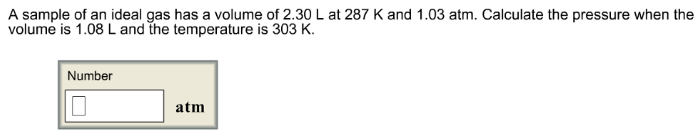# Problem: A sample of an ideal gas has a volume of 2.30 L at 287 K and 1.03 atm. Calculate the pressure when the volume is 1.08 L and the temperature is 303 K.

###### FREE Expert Solution###### Problem Details

A sample of an ideal gas has a volume of 2.30 L at 287 K and 1.03 atm. Calculate the pressure when the volume is 1.08 L and the temperature is 303 K.